Question

Four particles, one at each of the four corners of a square with 2.5-m-long edges, are connected by mass less rods

Four particles, one at each of the four corners of a square with 2.5-m-long edges, are connected by mass less rods. The masses of the particles are m1 = m3 = 2.0 kg and m2 = m4 = 7.0 kg. Find the moment of inertia of the system about the z axis.

Equation: I=M*L^2

M is the mass of the object.
I is the moment of inertia.
L is the length squared or radius squared.

I=(2.5^2(m2+m1))+ (2.5^2(m2+m3))
I= 6.25*(9)+6.25*(9)
I=112.5 kg*m^2

Earn Coins

Coins can be redeemed for fabulous gifts.

Similar Homework Help Questions
• Four particles at the corners of a square with a side length L=2.00m are connected by...

Four particles at the corners of a square with a side length L=2.00m are connected by massless rods. The particle masses are m1= m4=4.00kg and m2= m3 = 16.0 kg. Pairs of particles with equal masses are located at opposite corners of the square. Find the moment of inertia of the system about the z-axis that passes through a corner of the square where the particle has a mass of m=16.00kg.

• Four particles at the corners of a square with a side length L=4.00m are connected by...

Four particles at the corners of a square with a side length L=4.00m are connected by massless rods. The particle masses are m1= m4=2.00kg and m2= m3 = 16.0 kg. Pairs of particles with equal masses are located at opposite corners of the square. Find the moment of inertia of the system about the z-axis that passes through a corner of the square where the particle has a mass of m=16.00kg.

• Problem 9-43: Four particles at the corners of a square with a side length L-5.00m are connected by massless rods. The...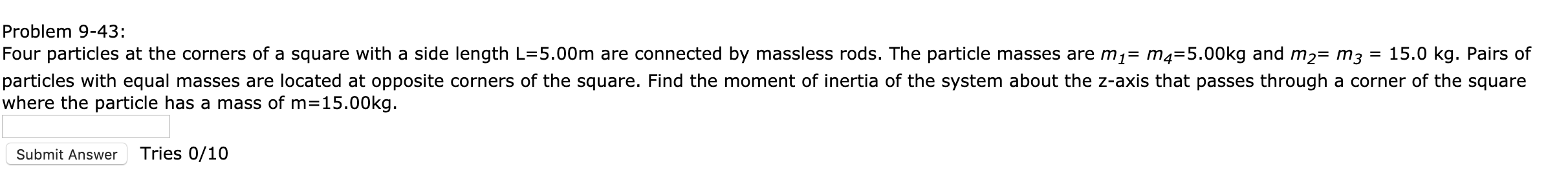Problem 9-43: Four particles at the corners of a square with a side length L-5.00m are connected by massless rods. The particle masses are m1= m4=5.00kg and m2= m3 particles with equal masses are located at opposite corners of the square. Find the moment of inertia of the system about the z-axis that passes through a corner of the square where the particle has a mass of m=15.00kg. 15.0 kg. Pairs of Tries 0/10 Submit Answer

• Four particles with masses 4 kg, 6 kg, 4 kg, and 6 kg are connected by rigid rods of negligible mass as shown.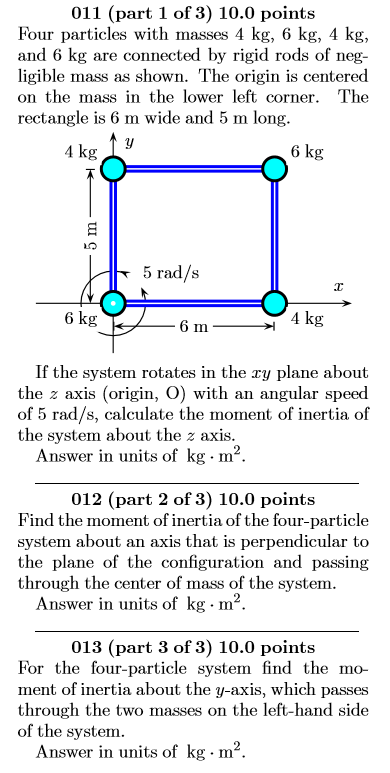011. Four particles with masses 4 kg, 6 kg, 4 kg, and 6 kg are connected by rigid rods of negligible mass as shown. The origin is centered on the mass in the lower left corner. The rectangle is 6 m wide and 5 m long. If the system rotates in the xy plane about the z axis (origin, O) with an angular speed of 5 rad/s, calculate the moment of inertia of the system about the z axis. 012. Find the...

• Four particles with equal masses M are connected by rods whose mass can be neglected. Find...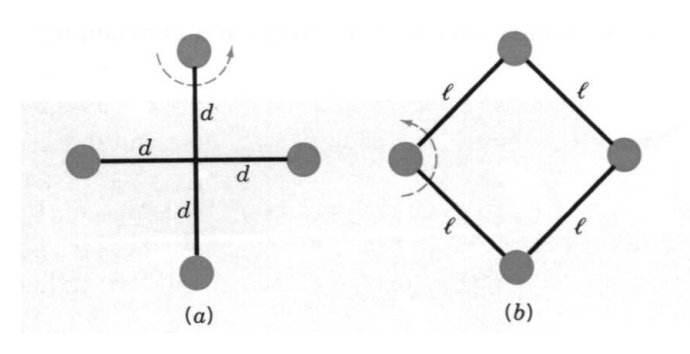Four particles with equal masses M are connected by rods whose mass can be neglected. Find the moment of inertia, about the indicated axis, of the following: (a) the cross in figure a, (b) the square in figure b.

• The four particles shown below are connected by rigid rods of negligible mass where y1 = 6.60 m.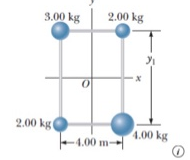The four particles shown below are connected by rigid rods of negligible mass where y1 = 6.60 m. The origin is at the center of the rectangle. The system rotates in the xy plane about the z axis with an angular speed of 6.40 rad/s. (a) Calculate the moment of inertia of the system about the z axis.(b) Calculate the rotational kinetic energy of the system.

• The four particles shown below are connected by rigid rods of negligible mass where y1 = 6.60 m.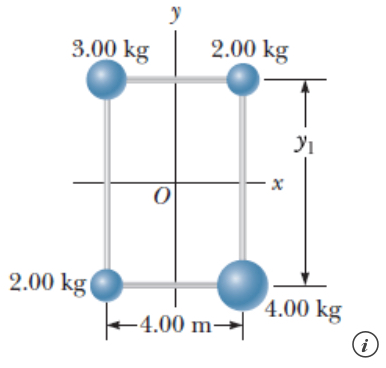The four particles shown below are connected by rigid rods of negligible mass where y1 = 6.60 m. The origin is at the center of the rectangle. The system rotates in the xy plane about the z axis with an angular speed of 5.40 rad/s. (a) Calculate the moment of inertia of the system about the z axis.(b) Calculate the rotational kinetic energy of the system.

• Four objects are held in position at the corners of a rectangle by light rods as...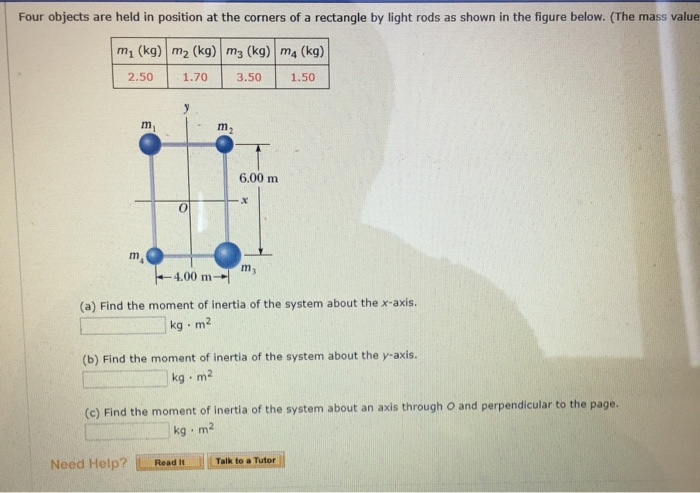Four objects are held in position at the corners of a rectangle by light rods as shown in the figure below. (The mass value m, (kg) mz (kg) mz (kg) ma (kg) 2.50 1.70 3.50 1.50 6.00 m 4.00 m m (a) Find the moment of inertia of the system about the x-axis. kg. m2 (b) Find the moment of inertia of the system about the y-axis. kg. m2 (c) Find the moment of inertia of the system about an...

• Four point masses, each of mass 1.1 kg are placed at the corners of a square...

Four point masses, each of mass 1.1 kg are placed at the corners of a square of side 1.6 m. A. Find the moment of inertia of this system about an axis that is perpendicular to the plane of the square and passes through one of the masses. B. If the system is set rotating about the above axis with kinetic energy of 195.0 J, what is the number of revolutions the system makes per minute

• Four masses are at corners of a rectangle connected by massless rods as shown in Figure...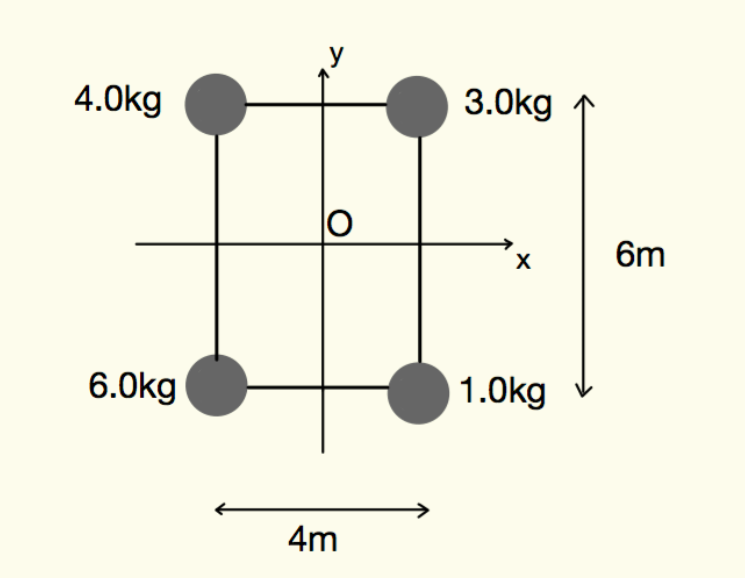Four masses are at corners of a rectangle connected by massless rods as shown in Figure 0.27. (i) What is the moment of inertia of the system when the axis of rotation is along the x-axis? (ii) What is the moment of inertia of the system when the axis of rotation is along the y-axis? (iIi) What is the moment of inertia of the system when the axis of rotation goes through point O and is perpendicular to the xy-plane....